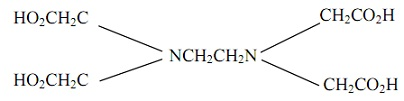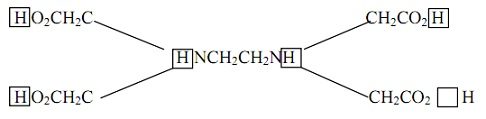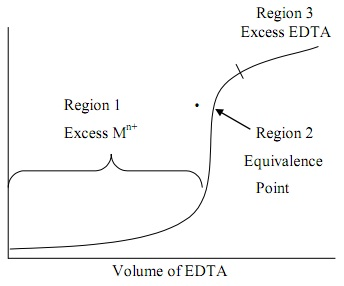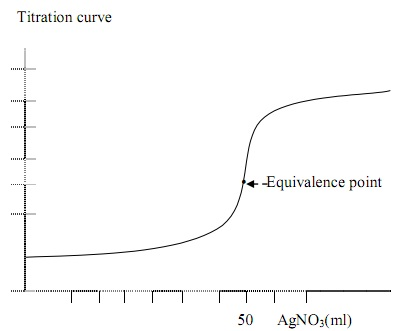#### Complexometric and Precipitation Titrations, Chemistry tutorial

Introduction:

Some of the metal ions form slightly soluble salts or slightly dissociated complexes. The formation of such complexes can be the base for accurate, more accurate and convenient titrimetric determination for these metal ions. Titration based on such complexes is termed as Complexometric titrimetric method.

Definition:

Complexometric titration is the analytical method that is employed to find out the larger number of metals which form soluble salt or slightly dissociated complexes.

Nearly all metals on the periodic table form complex with electron donating agent (that is, ligand) (example: O, N and S atoms) that are capable of satisfying the coordination number of such metal. The metals are Lewis acids (that is, electron accepting species); whereas the ligands are Lewis bases (that is, election pair donors).

Ligands:

Ligands are the Complexing agents which bind by the metals to form complexes. The number of ligand that complexes metals depends on the coordination number of the metal. Therefore there are mainly two kinds of ligands.

1) Monodentate Ligands: These are the ligands which bind the metal ion via only one atom (that is, the carbon atom). Example: CN- and NH3.

2) Multidentate Ligands: These are the kinds of ligands which attach to metal ion via more than one ligand atom. Illustration is EDTA (or ethylenediaminetetreacetic acid) termed as Chelating Ligand. ATP (adenosine triphosphate) is the other significant tetra dentate ligand.

Formation Constant:

Most of the ligands apart from, possibly, nitrilotriacetic acid (NTA), form complexes with metal ions in the stoichiometric 1:1 (ligand: metal ion) ratio in spite of the charge on the ion.

The equilibrium constant for the reaction of a metal ion by a ligand is termed as the formation constant Kf - It is as well termed as the stability constant Ks or Kstab

Kf = [Product]/[Reaction]

Example: Ag+ + 2NH3 ↔ Ag (NH3)2+

Kf = [Ag (NH3)2+]/[Ag+][NH3]2

As equilibria could as well be written in the reverse direction as dissociation. The constant is then termed as instability constant Ki, or the dissociation constant, Kd

Ag (NH3)2+ → Ag+ + 2(HN3)

Kd = [Ag+][NH3]2/[Ag(NH3)2]

Therefore, Kd = 1/Kf

EDTA Titrations:

EDTA is the most broadly employed chelator in the field of analytical chemistry, via direct titration or indirect sequence of reactions. It consists of a sharp end point corresponding to the stoichiometric complex formed. The ligand is termed as chelating agent, whereas the complex formed with metal ion is termed as chelate. EDTA is a hexaprotic system designated as H6Y2+ by the exact structure.Fig: EDTA Titrations

EDTA is the hexaprotic H6Y2+, as the number of acidic hydrogen atom lost on complete metal complex formation is six. The first four which are lost apply to carboxyl protons whereas the last two are of ammonium protons.Fig: EDTA-Hexaprotic

Though, the neutral acid is tetraprotic which is designates via H4Y, not all H4 referring to proton from carboxyl proton, having different PKa values.

EDTA consists of four PKa values corresponding to the step wise dissociation of the four protons

H4Y ↔ H+ + H3Y-         Ka1 = 1.0 x 10-2 [H+][H3Y-]/H4Y

H3Y- ↔ H+ + H2Y2-      Ka2 = 2.2 x 10-3 [H+][H2Y2-]/[H2Y-]

H2Y2- ↔ H+ + HY-3      Ka3 = 6.9 x 10-7 [H+] [HY-3]/[H2Y2-]

HY3- ↔ H+ + Y4-          Ka4 = 5.59 x 10-11 [H+][Y4-]/[HY3-]

Anion is the ligand species in complex formation, the complex made are markedly influenced by the pH. H4Y consists of low solubility in water, therefore the generally used reagent is disodium salt, (Na2H2Y2.2H2O).

The fraction [α] for each and every species is the fraction of EDTA in that form.

Conditional Formation Constant:

Due to the fucntionability of pH on the equilibrium, the fraction of EDTA is not all Y4- at pH beneath 10.24. Species like HY3-, HY2- predominate at lower pH.

Thus to conveniently deduce the fraction of free EDTA, there is the requirement employed for substitution and rearrangement as shown:

Replace   α Y4- [Y1] or α Y4- [EDTA] as [Y4]

Kf = [MYn-4]/[Mn+] α Y4-[Y-] or [MYA-4]/[Mn+] α Y4-[EDTA]

K1 = Conditional formation constant

Rearranging gives:

Kf α Y4- = K1 [MYn-4]/[Mn+][Y-] or [MYA-4]/[Mn+][EDTA]

This equation can be employed to compute the equilibrium concentration of the different species at a particular pH. It is as well termed as effective formation constant.

EDTA Titration Curve:

The concentration of the metal ion can be computed simply throughout the course of Complexometric titration of metal ion and EDTA in that chelating agent (titrant) is added to the sample having metal in (analyte). The titration is analogous to that of a strong acid metal ion and the weak base (EDTA).

The titration reaction is as follows:

Mn+ + EDTA ↔ MYN-4

Kf1 = α Y4- KFFig: EDTA Titration curve

If Kf1 is large, then the reaction is considered to be complete at each and every point of titration. The titration curve is generally divided into three stages.

1) Before equivalence point:

Every addition of EDTA is consumed fully at this phase; therefore there is surplus of Mn+ left. The concentration of free metal ion is equivalent to the concentration of excess, unreacted Mn+. The dissociation of MYn-4 is negligible.

2) At equivalence point:

The concentration of EDTA is precisely as that of metal ion in the solution. The solution is treated as if it is dissolving pure MYn-4

MYn-4 → Mn+ + EDTA

Therefore at equivalence point Mn+ = EDTA

3) After equivalence point:

Now there is surplus of EDTA whereas the whole metal ion has been virtually consumed and all metal ions in form of MYn-4. The concentration of free EDTA can be equated to the concentration of surplus EDTA added subsequent to the equivalence point.

Detecting the End Point:

There are processes comprised whenever trying to detect the end point in Complexometric titrations. Such methods comprise:

a) The utilization of metal ion indictor

b) Use of mercury electrode

c) Ion-selective electrode

d) Glass (pH) electrode

Though, the use of metal ion indicator seems the most convenient and proficient.

Metal Ion Indicator:

The metal-ion indicator is a compound that changes whenever it binds to a metal ion. This is significant to note that:

For a metal-ion indicator to be helpful, it should bind metal less strongly that EDTA does.

There are so many different kinds of metal - ion indicators that comprise Erichrome Black T, Calmagite, xylenol, murexide, pyridylazonephthol and so on. A typical illustration is using of Erichrome Black T in the titration of Mg2+ with EDTA.

Mg In   +  EDTA →    Mg EDTA  + In

(Red)     colourless   colourless    (Blue)

Most of the metal ion indicators are acid-base indicator.  Whenever the metal-indicator doesn't dissociate simply to discharge metal to EDTA to form metal-EDTA complex, the metal is stated to be blocked.

Though, the blocked EDTA can be titrated via back titration. Illustration, surplus EDTA (standard) can be added to Cu2+. The indicator is added and surplus EDTA is back-titrated with Mg2+.

EDTA Titration methods:

There are many kinds of titration methods comprised with EDTA. This is most likely due to large number of elements which can be titrated via EDTA. The techniques comprise:

1) Direct titration:

In this kind of titration, analyte is titrated with standard EDTA. The analyte is buffered to a suitable pH at which the conditional formation constant is larger and free indicator consists of a distinct colour. Addition of auxiliary complexing agent like ammonia, tartarate and citrate is added to prevent the metal ion from, precipitating in the absence of EDTA.

2)  Back-Titration:

It is a known excess of EDTA is added to the analyte. The surplus EDTA is then titrated by standard solution of a second metal ion.

Back titration is helpful if the metal ion precipitate in the absence of EDTA.

The metal ion employed in the back titration should not displace the analyte metal ion from its EDTA sample.

3) Displacement Titration:

It is a kind of titration in which the analyte is generally treated with surplus Mg (EDTA)2 to displace Mg2+, that is later titrated by standard EDTA. Displacement titration is frequently used whenever metal ions don't encompass satisfactory indicator.

Mn+ + MgY2- → MYn-4 + Mg2+

4) Indirect Titration:

Anions which form precipitate by certain metal ion can be analyzed by EDTA by indirect titration.

Illustration: SO4- precipitates by excess Ba2+ at pH 1.0. BaSO4 is filtered, washed and boiled by surplus EDTA at pH 10, to bring back Ba2+ into solution as Ba [EDTA]2-. The surplus EDTA is back titrated by Mg2+.

5) The use of Masking Agent:

It is employed to prevent the element from interfering in the analysis of the other element. Masked element can though be damasked after the analysis of element of interest via reacting with anion that consists of stronger affinity by the element masked, after which it is then titrated.

Precipitation Titration:

This kind of titration is very helpful in finding out the concentration of analyte that precipitates by the anion or titrant. It is helpful, provided that equilibrium is rapid and an appropriate means of detecting the end point is available.Fig: Precipitation Titration

Consider Cl- being titrated by AgNO3 that is identical to acid-base titration. Before the equivalence point, part of the Cl- is consumed via AgNO3 to precipitate the AgCl. The pH is found out by the remaining Cl- in the system.

At equivalence point, there is saturated solution with AgCl. The Cl- is approximately closely similar with AgNO3   added, whereas at points beyond the equivalence point, this is determined from the concentration of Ag+ and Ksp values. The smaller the Ksp, the bigger the break at equivalence point.

Detecting End Point:

The end point can be detected by either using of potentiometer by a suitable electrode.  Indicator can as well suitably be employed. There are two major kinds of indicators.

1) First type:

It forms a colored compound with the titrant whenever it is in surplus. Illustration:

a) In Mohr method for finding Cl- which is titrated by AgNO3, chromate (CrO42-), soluble salt is the indicator. This forms yellow solutions. Whenever Cl- precipitate is complete, the first excess Ag+ reacts by indicator to precipitate the red Ag2ClO4.

b) In Volhard Titration, F2+ (Ferrion) is added as indicator that forms soluble complex by the first excess of titrant.

Fe2+ + SCN → Fe (SCN)2+

2) Second type:

This kind of indicator is adsorption indicator. The indicator becomes adsorbed on the precipitate at the equivalence point. The colour of the indicator changes whenever it is adsorbed.

Illustration: Fajans method. Fluorescien is employed as an indicator for the halides at pH 7.

Tutorsglobe: A way to secure high grade in your curriculum (Online Tutoring)